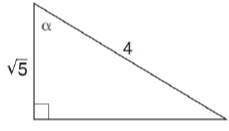Chapter 11.3, Problem 6EElementary Geometry For College St...

7th Edition
Alexander + 2 others
ISBN: 9781337614085

Solutions

Chapter
SectionElementary Geometry For College St...

7th Edition
Alexander + 2 others
ISBN: 9781337614085
Textbook Problem

In Exercises 5 to 10, find the value (or expression) for each of the six trigonometric ratios of angle α . Use the Pythagorean Theorem as needed.To determine

To find:

The value for each of the six trigonometric ratios of angle α.

Explanation

Given:

The given figure is

Definition used:

The six trigonometric ratios:

Calculation:

Let the value of the unknown side in the figure be x.

In a right triangle, (hypotenuse)2=(opposite)2+(adjacent)2

(4)2=x2+(5)216=x2+5165=x211=x211=x

Thus we have

From the figure, opposite=11, adjacent=5, hypotenuse=4

Still sussing out bartleby?

Check out a sample textbook solution.

See a sample solution

The Solution to Your Study Problems

Bartleby provides explanations to thousands of textbook problems written by our experts, many with advanced degrees!

Get Started

In Problems 1-6, find the absolute maxima and minima for on the interval .

Mathematical Applications for the Management, Life, and Social Sciences

A sample of n = 9 scores has X = 108. What is the sample mean?

Essentials of Statistics for The Behavioral Sciences (MindTap Course List)

Sketch the region in the xy-plane. 51. {(x, y) | 1 + x y 1 2x}

Single Variable Calculus: Early Transcendentals, Volume I

In Exercises 4144, determine whether the statement is true or false. 41. 3 20

Applied Calculus for the Managerial, Life, and Social Sciences: A Brief Approach

The polar equation of the conic with eccentricity 3 and directrix x = −7 is:

Study Guide for Stewart's Single Variable Calculus: Early Transcendentals, 8th